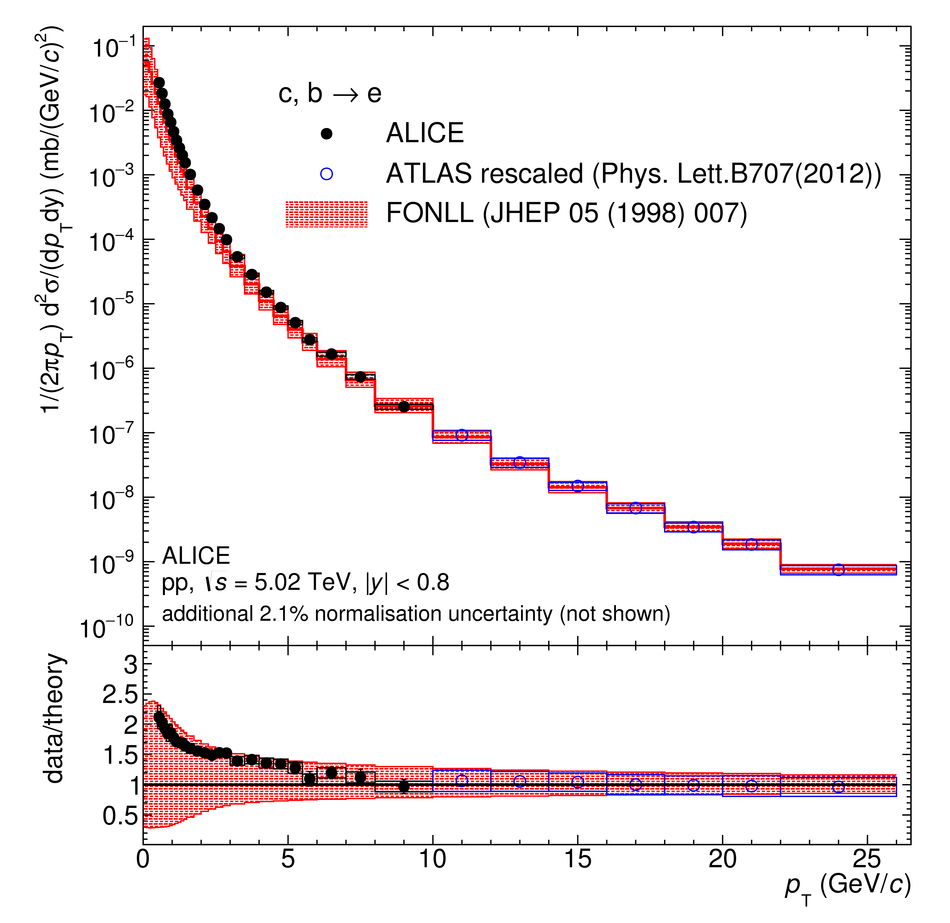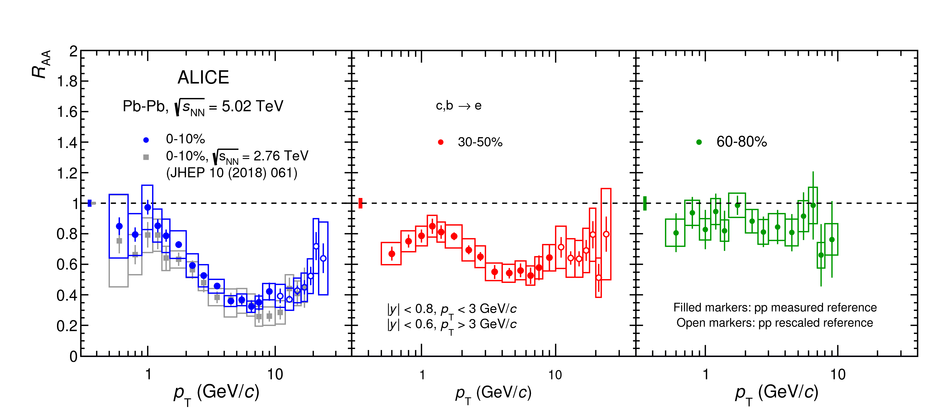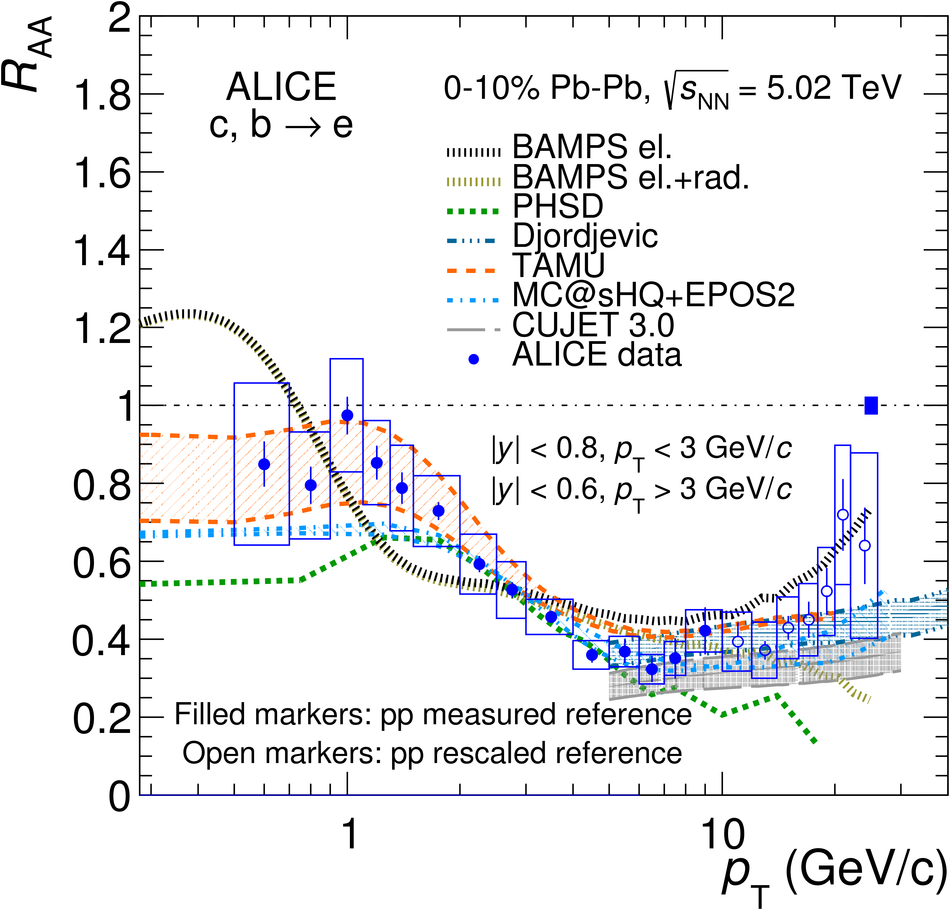# Measurement of electrons from semileptonic heavy-flavour hadron decays at midrapidity in pp and Pb-Pb collisions at $\sqrt{s_{\rm{NN}}}$ = 5.02 TeV

The differential invariant yield as a function of transverse momentum ($p_\mathrm{T}$) of electrons from semileptonic heavy-flavour hadron decays was measured at midrapidity in central (0-10%), semi-central (30-50%) and peripheral (60-80%) lead-lead (Pb-Pb) collisions at $\sqrt{s_{\mathrm{NN}}}=5.02\text{ TeV}$ in the $p_{\mathrm{T}}$ intervals 0.5-26 GeV/$c$ (0-10% and 30-50%) and 0.5-10 GeV/$c$ (60-80%). The production cross section in proton-proton (pp) collisions at $\sqrt{s}=5.02$ TeV was measured as well in $0.5<~p_\mathrm{T}<~10$ GeV/$c$ and it lies close to the upper band of perturbative QCD calculation uncertainties up to $p_\mathrm{T}=5$ GeV/$c$ and close to the mean value for larger $p_\mathrm{T}$. The modification of the electron yield with respect to what is expected for an incoherent superposition of nucleon-nucleon collisions is evaluated by measuring the nuclear modification factor $R_{\mathrm{AA}}$. The measurement of the $R_{\mathrm{AA}}$ in different centrality classes allows in-medium energy loss of charm and beauty quarks to be investigated. The $R_{\mathrm{AA}}$ shows a suppression with respect to unity at intermediate $p_\mathrm{T}$, which increases while moving towards more central collisions. Moreover, the measured $R_{\mathrm{AA}}$ is sensitive to the modification of the parton distribution functions (PDF) in nuclei, like nuclear shadowing, which causes a suppression of the heavy-quark production at low $p_\mathrm{T}$ in heavy-ion collisions at LHC.

Figures

## Figure 1

 $p_\mathrm{T}$-differential invariant production cross section of electrons from semileptonic heavy-flavour hadron decays in pp collisions at $\sqrt{s}=5.02$~TeV. The measurement is compared with the FONLL calculation . In the bottom panel, the ratios with respect to the central values of the FONLL calculation are shown. An additional 2.1\% normalisation uncertainty, due to the measurement of the minimum bias triggered cross section , is not shown in the results.## Figure 2

 $p_\mathrm{T}$-differential invariant yield in central (0--10\%), semi-central (30--50\%), and peripheral (60--80\%) Pb--Pb collisions at $\sqrt{s_\mathrm{NN}}=5.02$ TeV.## Figure 3

 Nuclear modification factor of electrons from semileptonic heavy-flavour hadron decays measured in the three centrality intervals in Pb--Pb collisions at $\sqrt{s_\mathrm{NN}}=5.02$ TeV.## Figure 4

 Nuclear modification factor of electrons semileptonic from heavy-flavour hadron decays measured in 0--10\% and 30--50\% centrality in Pb--Pb collisions at $\sqrt{s_\mathrm{NN}}=5.02$ TeV compared with model predictions .